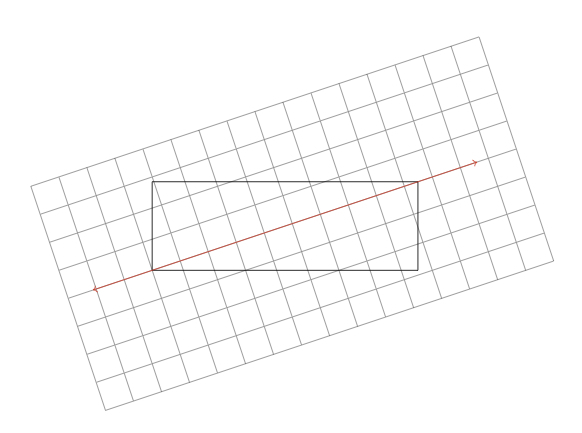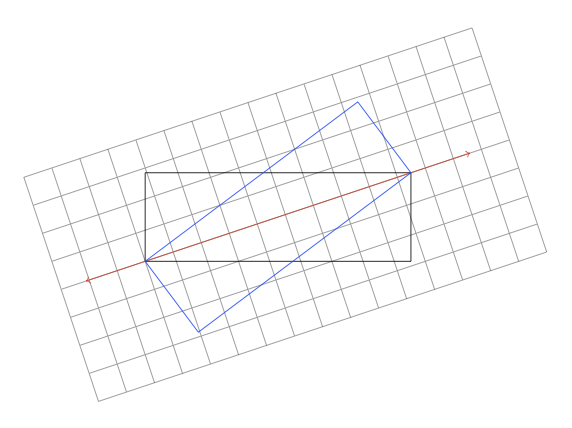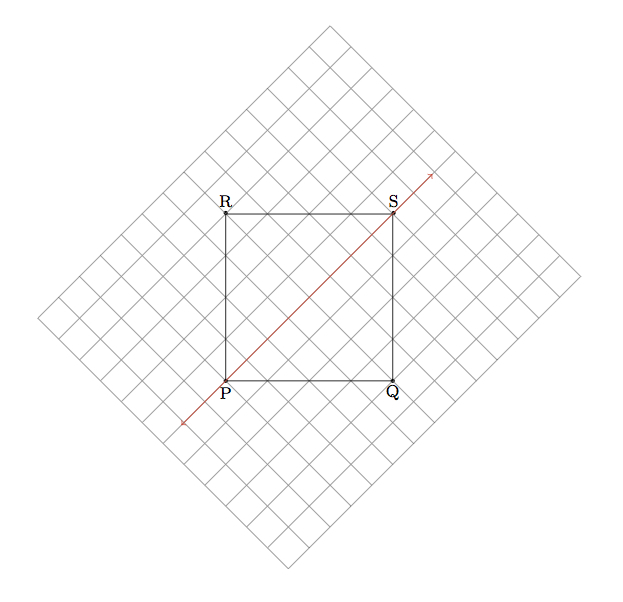# Reflecting a rectangle over a diagonal

Alignments to Content Standards: 8.G.A

1. Each picture below shows a rectangle with a line through a diagonal. For each picture, use the grid in the background to help draw the reflection of the rectangle over the line.
1.2.3.2. Suppose you have a rectangle where the line through the diagonal is a line of symmetry. Using what you know about reflections, explain why the rectangle must be a square.

## IM Commentary

The goal of this task is to give students experience applying and reasoning about reflections of geometric figures using their growing understanding of the properties of rigid motions. In the case of reflecting a rectangle over a diagonal, the reflected image is still a rectangle and it shares two vertices with the original rectangle. The examples show that the reflected image looks like a rotation of the original rectangle about its center point. Moreover, this "displacement" of the rectangle becomes smaller and smaller as the rectangle becomes closer to being a square. This leads naturally to the last part of the question: lines through a diagonal are lines of symmetry for a square (but not a non-square rectangle). Note that a line of symmetry can be thought of as a transformation that shows a figure is congruent to itself in a non-trivial way.

The task is intended for instructional purposes and assumes that students know the properties of rigid transformations described in 8.G.1. Note that the vertices of the rectangles in question do not fall exactly at intersections of the horizontal and vertical lines on the grid. This means that students need to approximate and this provides an extra challenge. Also providing a challenge is the fact that the grids have been drawn so that they are aligned with the diagonal of the rectangles rather than being aligned with the vertical and horizontal directions of the page. However, this choice of grid also makes it easier to reason about the reflections if they understand the descriptions of rigid motions indicated in 8.G.3.

Note that students study lines of symmetry in 4th grade, but only informally. While the 8th grade standards do not require students to reason as formally as they will in high school geometry, they are certainly able to reason more formally than they did in 4th grade. The solution to this task reflects this expectation. See 4.G Lines of symmetry for quadrilaterals for an example of what students might be expected to do in 4th grade.

## Solution

1. The reflections of each rectangle are pictured below in blue. In each case the reflected image shares two vertices with the original rectangle. This is because the line of reflection passes through two vertices and reflection over a line leaves all points on the line in their original position.

Notice that the reflected rectangle is, in each case, still a rectangle of the same size and shape as the original rectangle. Also notice that as the length and width of the rectangles become closer to one another, the two vertices are getting closer and closer to the vertices of the original rectangle. The case where the length and width of the rectangle are equal is examined in part (b).

For each of the pictures below, the same type of reasoning applies: the red line can be thought of as the $x$-axis of the grid. Reflecting over the $x$-axis does not change the $x$-coordinate of a point but it changes the sign of the $y$-coordinate. In the picture for (i), the rectangle vertex above the red line is just under three boxes above the red line. So its reflection is just under three units below the red line, with the same $x$ coordinate. Similarly in the picture for part (iii), the vertex of the rectangle below the red line is a little more than $3$ boxes below the red line: so its reflection will be on the same line through the $x$-axis but a little over three boxes above the red line. Similar reasoning applies to all vertices in the three pictures. Because reflections map line segments to line segments, knowing where the vertices of the rectangles map is enough to determine the reflected image of the rectangle.

1.2.3.2. Below is a picture of a quadrilateral $PQRS$ with the line $\overleftrightarrow{PS}$ containing the diagonal colored red. We assume $PQRS$ is mapped to itself by reflection over the $\overleftrightarrow{PS}$.Since $P$ and $S$ are on the line of reflection, they are mapped to themselves by the reflection. So the fact that the rectangle is mapped to itself means that $Q$ maps to $R$ and $R$ to $Q$. Since opposite sides of a rectangle are congruent we know that $|RS| = |PQ|$ and $|PR| = |QS|$. Reflection about line $\overleftrightarrow{PS}$ preserves line segments and sends segment $\overline{PR}$ to segment $\overline{PQ}$. Reflections preserve line segment lengths so $|PQ| = |PR|$. Putting our equalities together we find $$|RS| = |PQ| = |PR| = |QS|.$$ Since $PQRS$ is a rectangle whose four sides have the same length, it must be a square.